Ellipse - Path traced

Chapter 11 Class 11 Conic Sections
Concept wise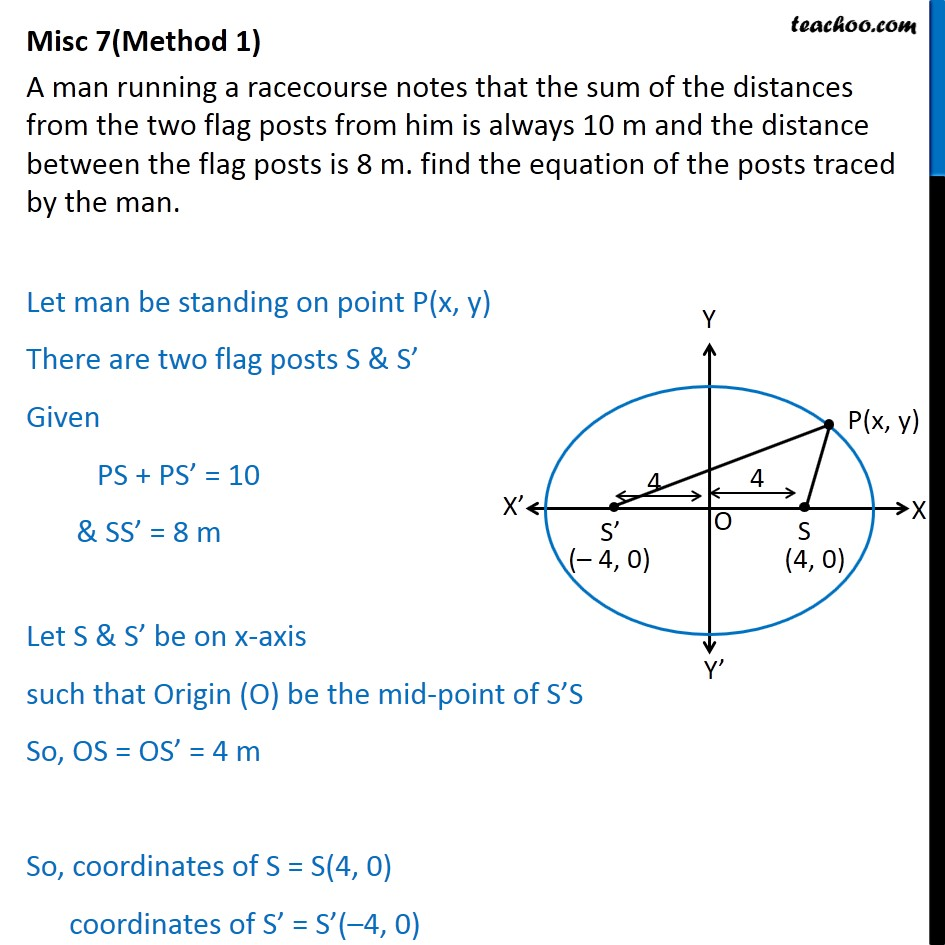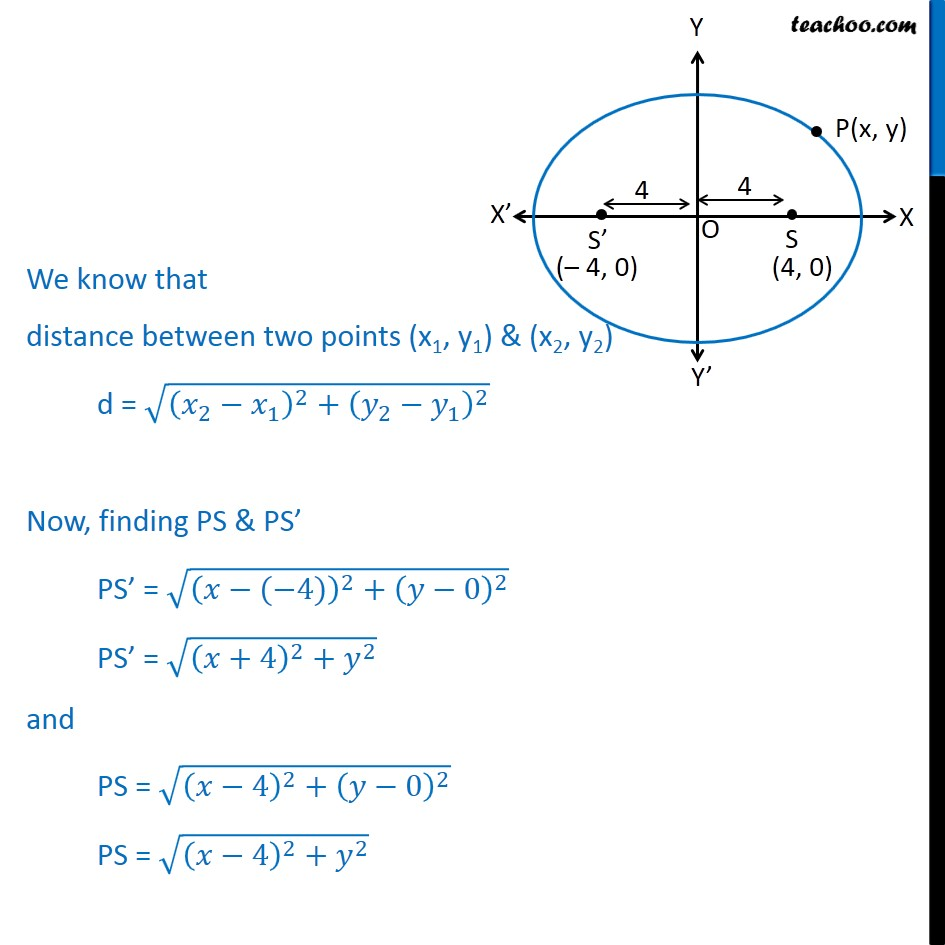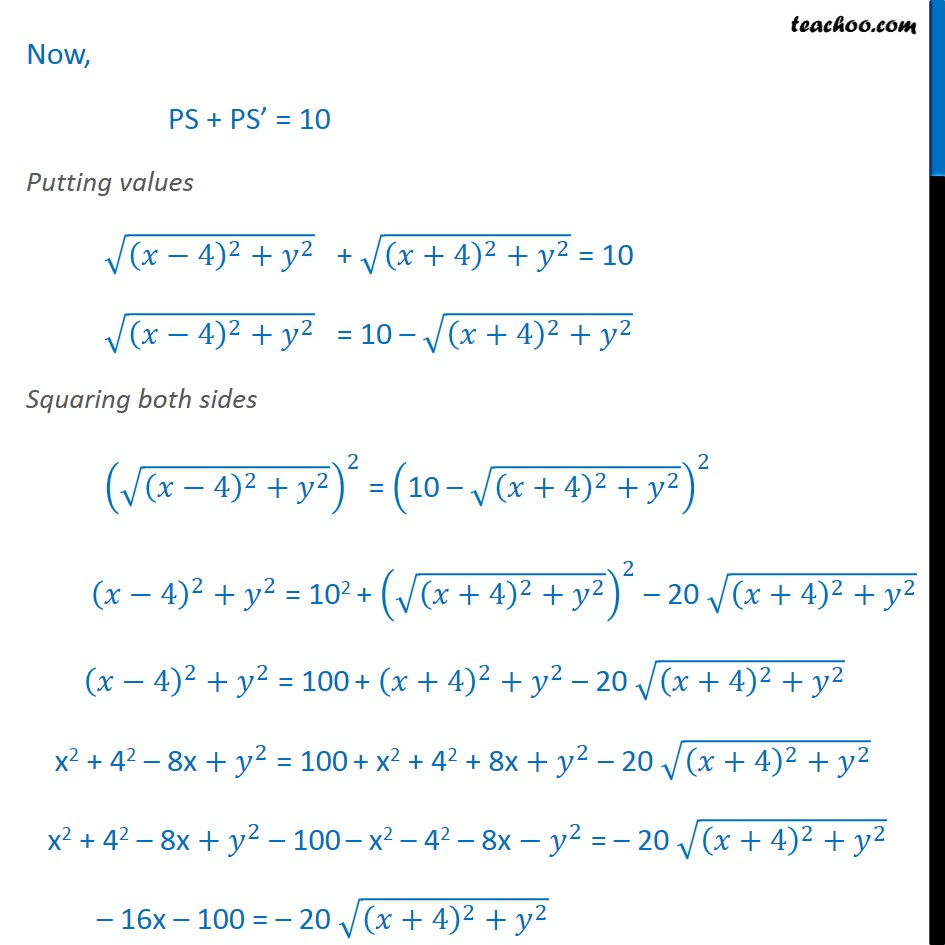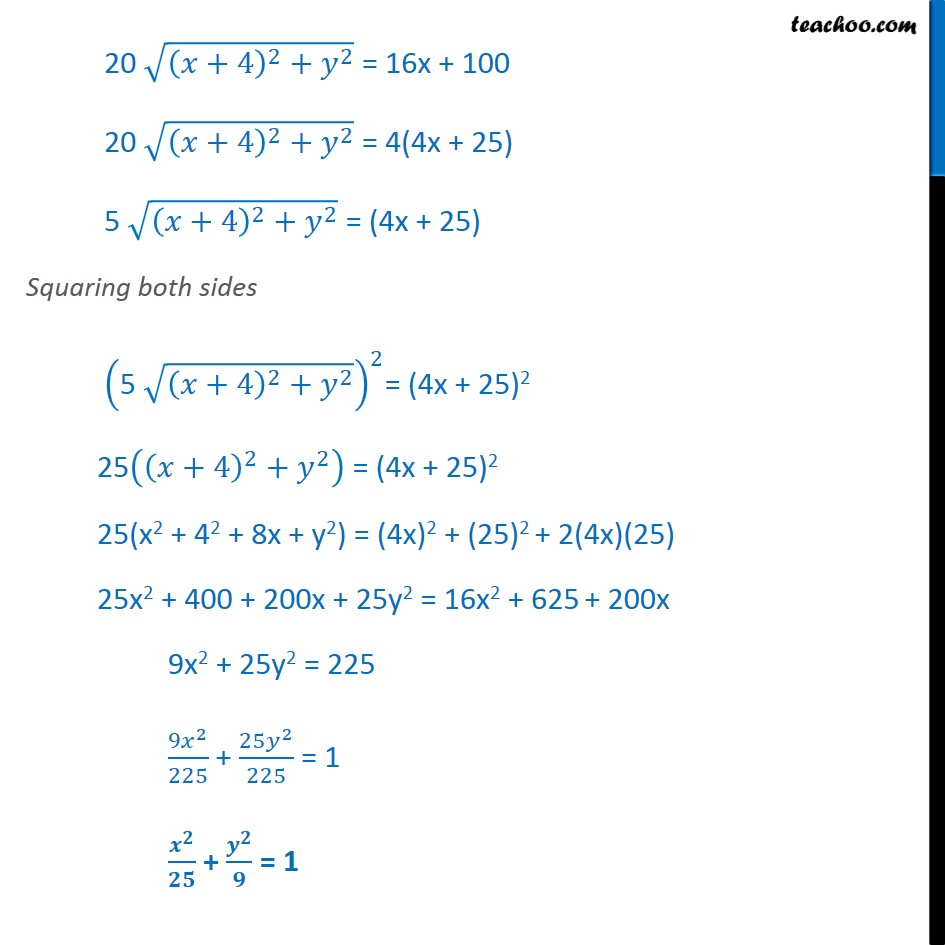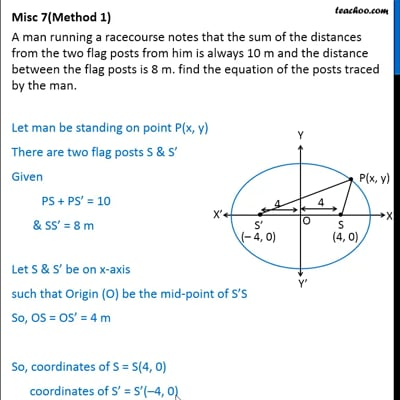This video is only available for Teachoo black users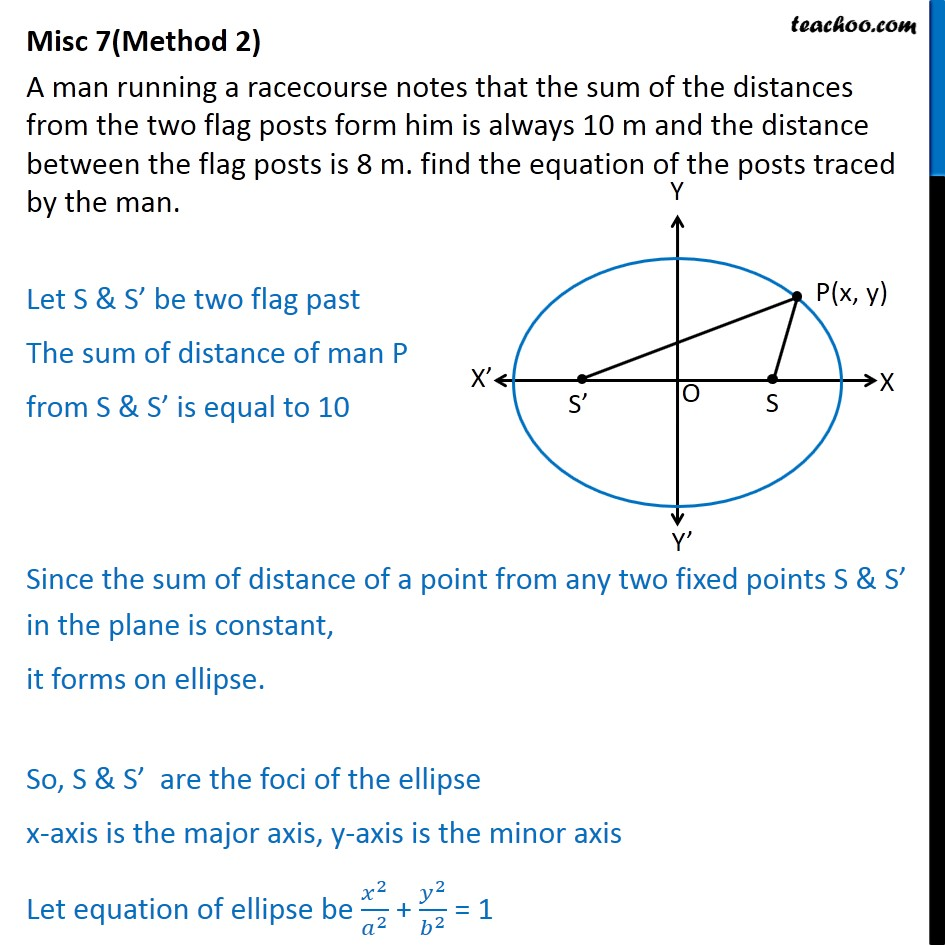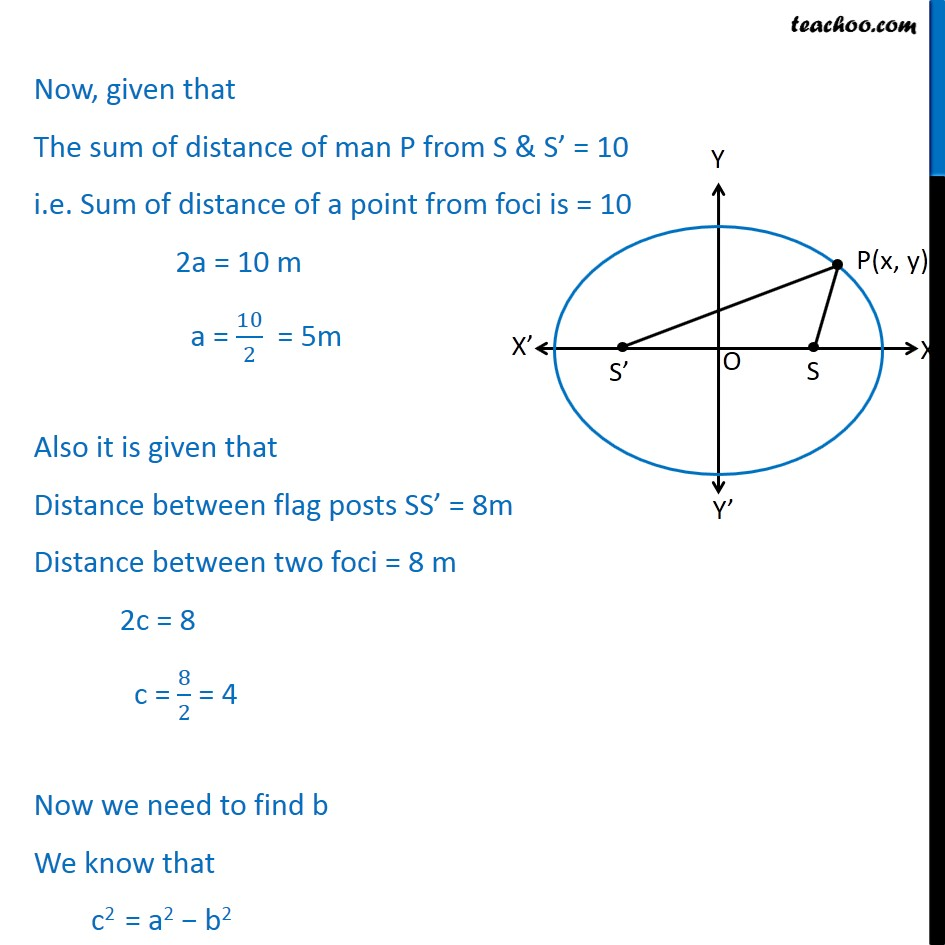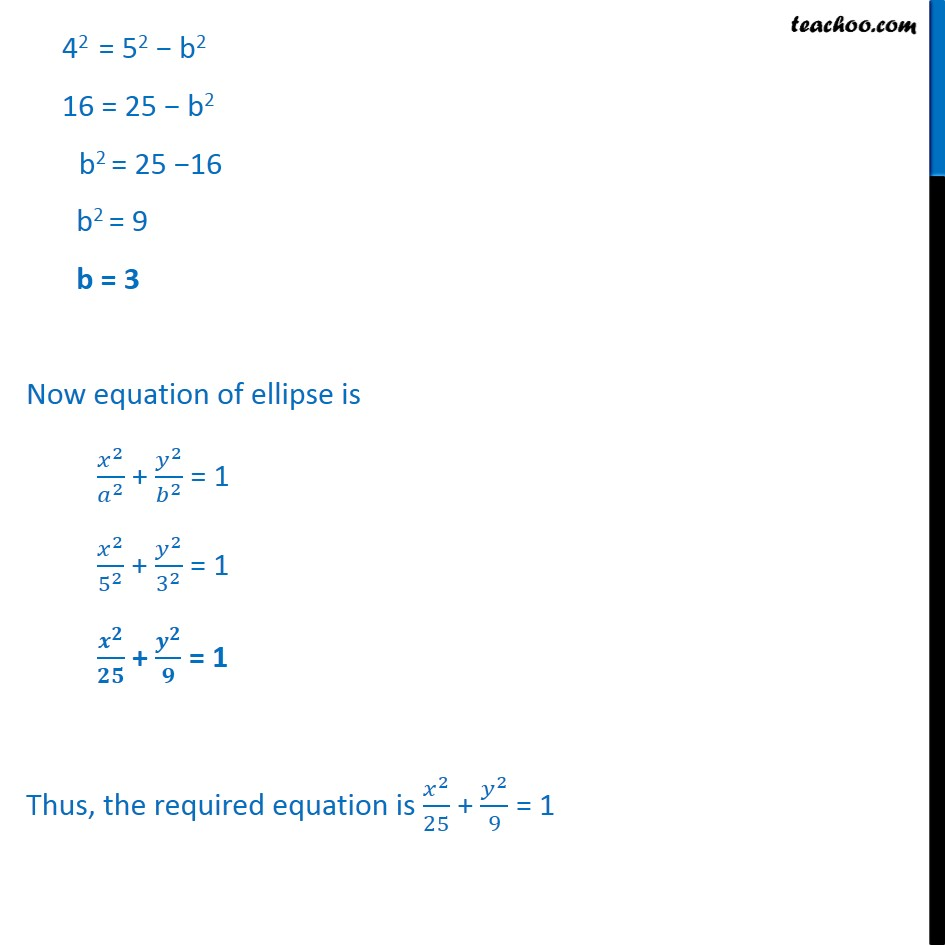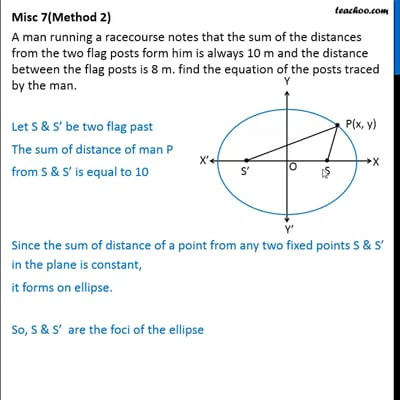This video is only available for Teachoo black users

Introducing your new favourite teacher - Teachoo Black, at only ₹83 per month

### Transcript

Misc 7(Method 1) A man running a racecourse notes that the sum of the distances from the two flag posts from him is always 10 m and the distance between the flag posts is 8 m. find the equation of the posts traced by the man. Let man be standing on point P(x, y) There are two flag posts S & S Given PS + PS = 10 & SS = 8 m Let S & S be on x-axis such that Origin (O) be the mid-point of S S So, OS = OS = 4 m So, coordinates of S = S(4, 0) coordinates of S = S ( 4, 0) We know that distance between two points (x1, y1) & (x2, y2) d = 2 1 2 + 2 1 2 Now, finding PS & PS PS = ( 4) 2 + 0 2 PS = +4 2 + 2 and PS = 4 2 + 0 2 PS = 4 2 + 2 Now, PS + PS = 10 Putting values 4 2 + 2 + +4 2 + 2 = 10 4 2 + 2 = 10 +4 2 + 2 Squaring both sides 4 2 + 2 2 = 10 +4 2 + 2 2 4 2 + 2 = 102 + +4 2 + 2 2 20 +4 2 + 2 4 2 + 2 = 100 + +4 2 + 2 20 +4 2 + 2 x2 + 42 8x + 2 = 100 + x2 + 42 + 8x + 2 20 +4 2 + 2 x2 + 42 8x + 2 100 x2 42 8x 2 = 20 +4 2 + 2 16x 100 = 20 +4 2 + 2 20 +4 2 + 2 = 16x + 100 20 +4 2 + 2 = 4(4x + 25) 5 +4 2 + 2 = (4x + 25) Squaring both sides 5 +4 2 + 2 2 = (4x + 25)2 25 +4 2 + 2 = (4x + 25)2 25(x2 + 42 + 8x + y2) = (4x)2 + (25)2 + 2(4x)(25) 25x2 + 400 + 200x + 25y2 = 16x2 + 625 + 200x 9x2 + 25y2 = 225 9 2 225 + 25 2 225 = 1 + = 1 Misc 7(Method 2) A man running a racecourse notes that the sum of the distances from the two flag posts form him is always 10 m and the distance between the flag posts is 8 m. find the equation of the posts traced by the man. Let S & S be two flag past The sum of distance of man P from S & S is equal to 10 Since the sum of distance of a point from any two fixed points S & S in the plane is constant, it forms on ellipse. So, S & S are the foci of the ellipse x-axis is the major axis, y-axis is the minor axis Let equation of ellipse be 2 2 + 2 2 = 1 Now, given that The sum of distance of man P from S & S = 10 i.e. Sum of distance of a point from foci is = 10 2a = 10 m a = 10 2 = 5m Also it is given that Distance between flag posts SS = 8m Distance between two foci = 8 m 2c = 8 c = 8 2 = 4 Now we need to find b We know that c2 = a2 b2 42 = 52 b2 16 = 25 b2 b2 = 25 16 b2 = 9 b = 3 Now equation of ellipse is 2 2 + 2 2 = 1 2 5 2 + 2 3 2 = 1 + = 1 Thus, the required equation is 2 25 + 2 9 = 1